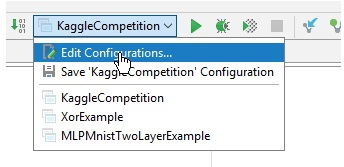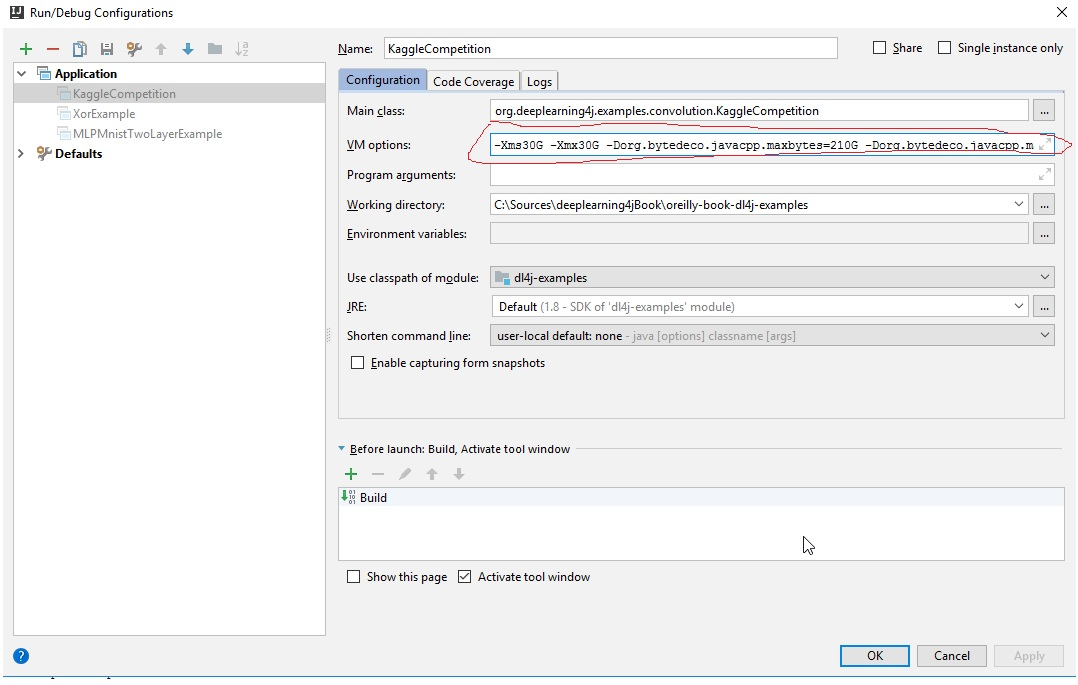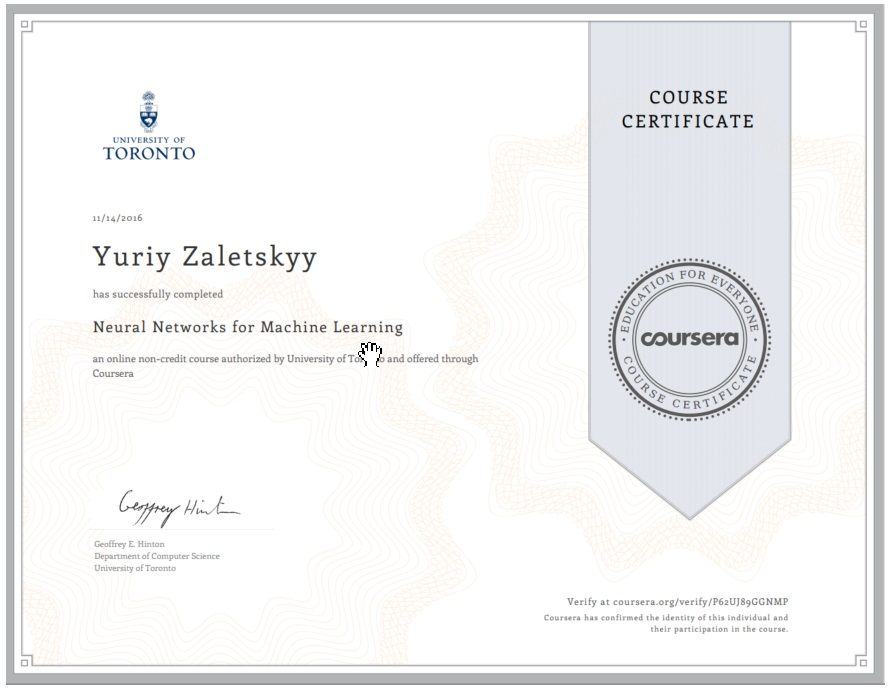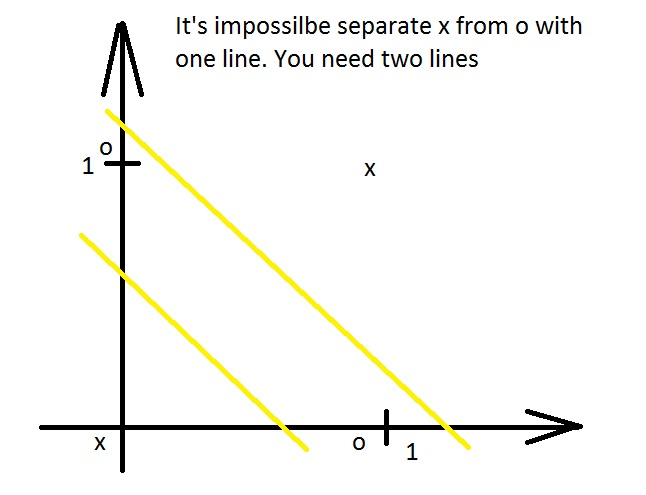Greetings everybody,

here I want to preserve for future usage my code which I've used to train model for one of Kagglecompetitions:

```  1 package org.deeplearning4j.examples.convolution;
2
4 import org.deeplearning4j.api.storage.StatsStorage;
5 import org.deeplearning4j.datasets.iterator.BaseDatasetIterator;
6 import org.deeplearning4j.datasets.iterator.FloatsDataSetIterator;
7 import org.deeplearning4j.datasets.iterator.impl.ListDataSetIterator;
8 import org.deeplearning4j.datasets.iterator.impl.MnistDataSetIterator;
9 import org.deeplearning4j.eval.Evaluation;
10 import org.deeplearning4j.nn.api.OptimizationAlgorithm;
11 import org.deeplearning4j.nn.conf.MultiLayerConfiguration;
12 import org.deeplearning4j.nn.conf.NeuralNetConfiguration;
13 import org.deeplearning4j.nn.conf.Updater;
14 import org.deeplearning4j.nn.conf.inputs.InputType;
15 import org.deeplearning4j.nn.conf.layers.ConvolutionLayer;
16 import org.deeplearning4j.nn.conf.layers.DenseLayer;
17 import org.deeplearning4j.nn.conf.layers.OutputLayer;
18 import org.deeplearning4j.nn.conf.layers.SubsamplingLayer;
19 import org.deeplearning4j.nn.multilayer.MultiLayerNetwork;
20 import org.deeplearning4j.nn.weights.WeightInit;
21 import org.deeplearning4j.optimize.listeners.ScoreIterationListener;
22 import org.deeplearning4j.ui.api.UIServer;
23 import org.deeplearning4j.ui.stats.StatsListener;
24 import org.deeplearning4j.ui.storage.InMemoryStatsStorage;
25 import org.deeplearning4j.util.ModelSerializer;
26 import org.jetbrains.annotations.NotNull;
27 import org.nd4j.linalg.activations.Activation;
28 import org.nd4j.linalg.api.ndarray.INDArray;
29 import org.nd4j.linalg.dataset.DataSet;
30 import org.nd4j.linalg.dataset.api.iterator.DataSetIterator;
31 import org.nd4j.linalg.factory.Nd4j;
32 import org.nd4j.linalg.lossfunctions.LossFunctions;
33 import org.slf4j.Logger;
34 import org.slf4j.LoggerFactory;
35
36 import java.io.*;
37 import java.text.SimpleDateFormat;
38 import java.util.*;
39 import java.util.function.Consumer;
40 import java.util.stream.Collectors;
41
42 import org.apache.commons.io.FilenameUtils;
43
44 /**
45  * Created by Yuriy Zaletskyy
46  */
47 public class KaggleCompetition {
48     private static final Logger log = LoggerFactory.getLogger(KaggleCompetition.class);
49
50     public static void main(String[] args) throws Exception {
51
52         int nChannels = 8; // Number of input channels
53         int outputNum = 17; // The number of possible outcomes
54         int batchSize = 50; // Test batch size
55         int nEpochs = 1001; // Number of training epochs
56         int iterations = 1; // Number of training iterations
57         int seed = 123; //
58         int learningSetSize = 800;
59         int x = 256;
60         int y = 330;
61         int z = 8;
62         int sizeInt = 4;
63         int sizeOfOneVideo = x * y * z;
64         int numberOfZones = 17;
65
66         String labelsFileName = "d:\\Kaggle\\stage1_labels.csv";
68
69         String folderName = "d:\\Kaggle\\stage1_bins_resized\\";
70
71         INDArray input = Nd4j.zeros(learningSetSize + 1, sizeOfOneVideo);
72         INDArray outputKaggle = Nd4j.zeros(learningSetSize +1, numberOfZones);
73         File folder = new File(folderName);
74         List<String> fileNames = new ArrayList<String>(500);
75
76         GetFileNames(folder, fileNames);
77
78         int rowNumber = 0;
79         Date timeMarker = new Date();
80         SimpleDateFormat sdf = new SimpleDateFormat("yyyy-MM-dd HH:mm:ss");
81         System.out.println("Before reading files " + sdf.format(timeMarker));
82         for(String fileName : fileNames)
83         {
84             InputStream inputStream = null;
85             DataInputStream dataInputStream = null;
86
87             dataInputStream = new DataInputStream(new FileInputStream(fileName));
88
89             LittleEndianDataInputStream lendian = new LittleEndianDataInputStream(dataInputStream);
90
91             List<Float> listOfFloats = new ArrayList<Float>();
92
93             int fileSize = x * y * z * sizeInt;
94
95             byte[] fileContent = new byte[fileSize];
97
99
100             lendian.close();
101             dataInputStream.close();
102
103             File f = new File(fileName);
104             String containsFilter = FilenameUtils.removeExtension(f.getName());
105             List<String> outputStrings = labels.stream().filter(a -> a.contains(containsFilter))
106                 .collect(Collectors.toList());
107
108
109             int indexToTurnOn = getIndexToTurnOn(outputStrings);
110
111             float[] zoneOut = new float;
112
113             for(int i = 0; i < 17; i++)
114             {
115                 zoneOut[i] = 0.0f;
116                 if(i == indexToTurnOn)
117                 {
118                     zoneOut[i] = 1.0f;
119                 }
120             }
121
122             float[] inputRow = new float[listOfFloats.size()];
123             int j = 0;
124             for(Float ff: listOfFloats)
125             {
126                 inputRow[j++] = (ff != null ? ff: Float.NaN);
127             }
128
129             input.putRow(rowNumber, Nd4j.create(inputRow));
130
131             outputKaggle.putRow(rowNumber, Nd4j.create(zoneOut));
132
133             if(rowNumber > learningSetSize - 1)
134             {
135                 break;
136             }
137             rowNumber++;
138         }
139
140         timeMarker = new Date();
141         System.out.println("After reading files " + sdf.format(timeMarker));
142
144         DataSet dsKaggleAll = new DataSet(input, outputKaggle);
145
146         List<DataSet> listDs = dsKaggleAll.asList();
147
148         Random rng = new Random(seed);
149         Collections.shuffle(listDs,rng);
150
151         DataSetIterator dsKaggle = new ListDataSetIterator(listDs, batchSize);
152         log.info("Build model....");
153         MultiLayerConfiguration conf = new NeuralNetConfiguration.Builder()
154                 .seed(seed)
155                 .iterations(iterations) // Training iterations as above
156                 .regularization(true).l2(0.001)
157                 /*
158                     Uncomment the following for learning decay and bias
159                  */
160                 .learningRate(.0001).biasLearningRate(0.02)
161                 //.learningRateDecayPolicy(LearningRatePolicy.Inverse).lrPolicyDecayRate(0.001).lrPolicyPower(0.75)
162                 .weightInit(WeightInit.XAVIER)
164                 .updater(Updater.NESTEROVS).momentum(0.9)
165                 .list()
166                 .layer(0, new ConvolutionLayer.Builder(5, 5)
167                         //nIn and nOut specify depth. nIn here is the nChannels and nOut is the number of filters to be applied
168                         .nIn(nChannels)
169                         .stride(1, 1)
170                         .nOut(40)
171                         .activation(Activation.IDENTITY)
172                         .build())
173                 .layer(1, new SubsamplingLayer.Builder(SubsamplingLayer.PoolingType.MAX)
174                         .kernelSize(2,2)
175                         .stride(2,2)
176                         .build())
177                 .layer(2, new ConvolutionLayer.Builder(5, 5)
178                         //Note that nIn need not be specified in later layers
179                         .stride(1, 1)
180                         .nOut(100)
181                         .activation(Activation.IDENTITY)
182                         .build())
183                 .layer(3, new SubsamplingLayer.Builder(SubsamplingLayer.PoolingType.MAX)
184                         .kernelSize(2,2)
185                         .stride(2,2)
186                         .build())
187                 .layer(4, new DenseLayer.Builder().activation(Activation.RELU)
188                         .nOut(500).build())
189                 .layer(5, new OutputLayer.Builder(LossFunctions.LossFunction.NEGATIVELOGLIKELIHOOD)
190                         .nOut(outputNum)
191                         .activation(Activation.SOFTMAX)
192                         .build())
193                 .setInputType(InputType.convolutionalFlat(x,y,z)) //See note below
194                 .backprop(true).pretrain(true).build();
195
196
197         MultiLayerNetwork model = new MultiLayerNetwork(conf);
198         model.init();
199
200
201         log.info("Train model....");
202
203         //model = ModelSerializer.restoreMultiLayerNetwork("d:\\Kaggle\\models\\2017-12-1902_33_38.zip");
204         model.setListeners(new ScoreIterationListener(1));
205
206         //Initialize the user interface backend
207         UIServer uiServer = UIServer.getInstance();
208
209         //Configure where the network information (gradients, score vs. time etc) is to be stored. Here: store in memory.
210         StatsStorage statsStorage = new InMemoryStatsStorage();         //Alternative: new FileStatsStorage(File), for saving and loading later
211
212         //Attach the StatsStorage instance to the UI: this allows the contents of the StatsStorage to be visualized
213         uiServer.attach(statsStorage);
214
215         //Then add the StatsListener to collect this information from the network, as it trains
216         model.setListeners(new StatsListener(statsStorage));
217
218         for( int i=0; i< nEpochs; i++ ) {
219             dsKaggle.reset();
220             model.fit(dsKaggle);
221             log.info("*** Completed epoch {} ***", i);
222
223             //log.info("Evaluate model....");
224             //Evaluation eval = new Evaluation(outputNum);
225
226             timeMarker = new Date();
227             String fileName = "d:\\Kaggle\\models\\" + (sdf.format(timeMarker) + ".zip")
228                 .replace(" ", "")
229                 .replace(":", "_");
230             ModelSerializer.writeModel(model,fileName,true);
231         }
232         log.info("****************Example finished********************");
233     }
234
235      private static void ReadFromFile(List<Float> listOfFloats, int fileSize, byte[] fileContent) {
236         float min, max;
237         min = Float.MAX_VALUE;
238         max = Float.MIN_VALUE;
239         for(int i = 0; i < fileSize; i+=4)
240         {
241             int valueInt = (fileContent[i + 3]) << 24 |
242                 (fileContent[i + 2] &0xff) << 16 |
243                 (fileContent[i + 1] &0xff) << 8 |
244                 (fileContent[i]&0xff);
245             float value = Float.intBitsToFloat(valueInt);
246             if(value > max)
247             {
248                 max = value;
249             }
250             if(value < min)
251             {
252                 min = value;
253             }
255         }
256
257         for(int i = 0; i < listOfFloats.size(); i++)
258         {
259             float normalized = ( listOfFloats.get(i) - min ) / (max - min);
260             listOfFloats.set(i, normalized);
261         }
262     }
263
264     private static int getIndexToTurnOn(List<String> outputStrings) {
265         int indexToTurnOn = 0;
266         for(String outString : outputStrings)
267         {
268             String[] strings = outString.split("_");
269             String[] secondPart = strings.split(",");
270
271             String zoneName = secondPart.replace("Zone", "");
272             String zoneValue = secondPart;
273
274             if(zoneValue.equals("1"))
275             {
276                 indexToTurnOn = Integer.parseInt(zoneName);
277                 break;
278             }
279         }
280         return indexToTurnOn;
281     }
282
283     public static List<String> ReadCsvFile(String csvFile )
284     {
285         List<String> result = new ArrayList<String>();
286
288         String line = "";
289         String cvsSplitBy = ",";
290
291         try {
292
294             while ((line = br.readLine()) != null) {
296             }
297
298         } catch (FileNotFoundException e) {
299             e.printStackTrace();
300         } catch (IOException e) {
301             e.printStackTrace();
302         } finally {
303             if (br != null) {
304                 try {
305                     br.close();
306                 } catch (IOException e) {
307                     e.printStackTrace();
308                 }
309             }
310         }
311
312         return result;
313     }
314
315     private static void GetFileNames(File folder, List<String> fileNames) {
316         File[] listOfFiles = folder.listFiles();
317         for(File file : listOfFiles)
318         {
319             if(file.isFile())
320             {
322             }
323         }
324     }
325 }
326
327 ```

Among different features this code demonstrates mostly how to read some data, how to create network, save state of network in file, load state of network from file, and how to execute learning. Also this code presents way for monitoring network learning success via url http://localhost:9000

Hello everybody,

today I want to document one simple feature of Deeplearning4j library. Recently I had an assignment to feed into neural network for Deeplearning4j.

If your learning set is not big ( later I'll explain what big means ) then you can put all your data into INDArray and then based on that you can create DataSet. Take a look at fragments of XorExample.java:

```1         // list off input values, 4 training samples with data for 2
2         // input-neurons each
3         INDArray input = Nd4j.zeros(4, 2);
4
5         // correspondending list with expected output values, 4 training samples
6         // with data for 2 output-neurons each
7         INDArray labels = Nd4j.zeros(4, 2);```

Above Deeplearning4j team just reserved some small memory for learning.

Next goes filling information:

`// create first dataset// when first input=0 and second input=0input.putScalar(new int[]{0, 0}, 0);input.putScalar(new int[]{0, 1}, 0);// then the first output fires for false, and the second is 0 (see class// comment)labels.putScalar(new int[]{0, 0}, 1);labels.putScalar(new int[]{0, 1}, 0);// when first input=1 and second input=0input.putScalar(new int[]{1, 0}, 1);input.putScalar(new int[]{1, 1}, 0);// then xor is true, therefore the second output neuron fireslabels.putScalar(new int[]{1, 0}, 0);labels.putScalar(new int[]{1, 1}, 1);// same as aboveinput.putScalar(new int[]{2, 0}, 0);input.putScalar(new int[]{2, 1}, 1);labels.putScalar(new int[]{2, 0}, 0);labels.putScalar(new int[]{2, 1}, 1);// when both inputs fire, xor is false again - the first output should// fireinput.putScalar(new int[]{3, 0}, 1);input.putScalar(new int[]{3, 1}, 1);labels.putScalar(new int[]{3, 0}, 1);labels.putScalar(new int[]{3, 1}, 0);`

After that they create DataSet with all inputs, outputs:

`// create dataset objectDataSet ds = new DataSet(input, labels);`

I will skip neural network creation and configuration because purpose of this post is just explain about locating in memory learning set.

What is big?

As I mentioned initially what is big amount of data in Deeplearning4j. I'll explain with example. RAM amount on my server is 256 Gb. Let's mark it with variable ramAmount.

I want to feed into memory 800 files, 2703360 bytes each. In total they will take 800 * 2703360 ~ 2 Gb.

But when I applied Xor approach to mine dataset I've continiously got following error message:

Exception in thread "main" java.lang.IllegalArgumentException: Length is >= Integer.MAX_VALUE: lengthLong() must be called instead
at org.nd4j.linalg.api.ndarray.BaseNDArray.length(BaseNDArray.java:4203)
at org.nd4j.linalg.api.ndarray.BaseNDArray.init(BaseNDArray.java:2067)
at org.nd4j.linalg.api.ndarray.BaseNDArray.<init>(BaseNDArray.java:173)
at org.nd4j.linalg.cpu.nativecpu.NDArray.<init>(NDArray.java:70)
at org.nd4j.linalg.cpu.nativecpu.CpuNDArrayFactory.create(CpuNDArrayFactory.java:262)
at org.nd4j.linalg.factory.Nd4j.create(Nd4j.java:3911)
at org.nd4j.linalg.api.ndarray.BaseNDArray.create(BaseNDArray.java:1822)

as far as I grasp from mine conversations with support Deeplearning4j attempts to do the following: create one dimensional array which will be executed on all processors ( or video cards ). In my case it wasn possible only and only when my learning set was not 800, but something around 80. That is far less then waht I wanted to use for learning.

How to deal with big data set?

After realizing problem I had again dig deeper into Deeplearning4j samples. I found very useful sample of RegressionSum. There they create data set with help of function getTrainingData. Below goes source code of it:

``` 1 private static DataSetIterator getTrainingData(int batchSize, Random rand){
2         double [] sum = new double[nSamples];
3         double [] input1 = new double[nSamples];
4         double [] input2 = new double[nSamples];
5         for (int i= 0; i< nSamples; i++) {
6             input1[i] = MIN_RANGE + (MAX_RANGE - MIN_RANGE) * rand.nextDouble();
7             input2[i] =  MIN_RANGE + (MAX_RANGE - MIN_RANGE) * rand.nextDouble();
8             sum[i] = input1[i] + input2[i];
9         }
10         INDArray inputNDArray1 = Nd4j.create(input1, new int[]{nSamples,1});
11         INDArray inputNDArray2 = Nd4j.create(input2, new int[]{nSamples,1});
12         INDArray inputNDArray = Nd4j.hstack(inputNDArray1,inputNDArray2);
13         INDArray outPut = Nd4j.create(sum, new int[]{nSamples, 1});
14         DataSet dataSet = new DataSet(inputNDArray, outPut);
15         List<DataSet> listDs = dataSet.asList();
16         Collections.shuffle(listDs,rng);
17         return new ListDataSetIterator(listDs,batchSize);
18
19     }```

As you can see from the presented code, you need to

1. create one or more input arrays.
2. Create output array.
3. if you created more then one input arrays then you need to merge them in one array
4. Create DataSet that has inputs array and outputs array
5. Shuffle (  as usually this improves learning )
6. Return ListDataSetIterator

Configure memory for class in intellij idea

If you have hope that adventures with memory were completed I need to disappoint you. There were not. Next step that is needed for Deeplearning4j is configuration of available memory for particular class. Initially I got an impression that this can be done via edition vmoptions file of  In intellij idea. But that assumption is wrong. You'll need to configure memory for particular class like this:

1. select your class and choose Edit Configurations:2. Set some memory like presented at screenshot:IN my case I've used following line for memory:

-Xms30G -Xmx30G -Dorg.bytedeco.javacpp.maxbytes=210G -Dorg.bytedeco.javacpp.maxphysicalbytes=210G

Keep in mind that parameters -Dorg.bytedeco.javacpp.maxbytes should be equal to -Dorg.bytedeco.javacpp.maxphysicalbytes.

One more final detail to keep in mind, you'll also will need to think about parameter batchsize that you feed into neural network while configuring MultiLayerNetwork.

Hello everybody,

today I want to write a short note about normalization for neural networks.

So, first goes formula how to normalize input in range [0, 1] ( taken from here ):Another good for me example is going below ( taken from here ):

```p = [4 4 3 3 4;
2 1 2 1 1;
2 2 2 4 2];
```
```a = min(p(:));
b = max(p(:));
ra = 0.9;
rb = 0.1;
pa = (((ra-rb) * (p - a)) / (b - a)) + rb;```

In this example ra stands for maximum value of range, rb stands for minimum value of range that we want to make.

Hi,

I need to document my first implementation of learning algorithm for usage of neural networks for training

```package org.deeplearning4j.examples.recurrent;

import org.deeplearning4j.datasets.iterator.impl.ListDataSetIterator;
import org.deeplearning4j.nn.api.Layer;
import org.deeplearning4j.nn.api.OptimizationAlgorithm;
import org.deeplearning4j.nn.conf.BackpropType;
import org.deeplearning4j.nn.conf.MultiLayerConfiguration;
import org.deeplearning4j.nn.conf.NeuralNetConfiguration;
import org.deeplearning4j.nn.conf.Updater;
import org.deeplearning4j.nn.conf.layers.DenseLayer;
import org.deeplearning4j.nn.conf.layers.GravesLSTM;
import org.deeplearning4j.nn.conf.layers.OutputLayer;
import org.deeplearning4j.nn.conf.layers.RnnOutputLayer;
import org.deeplearning4j.nn.multilayer.MultiLayerNetwork;
import org.deeplearning4j.nn.weights.WeightInit;
import org.deeplearning4j.optimize.listeners.ScoreIterationListener;
import org.deeplearning4j.util.ModelSerializer;
import org.nd4j.linalg.api.ndarray.INDArray;
import org.nd4j.linalg.dataset.DataSet;
import org.nd4j.linalg.dataset.api.iterator.DataSetIterator;
import org.nd4j.linalg.factory.Nd4j;
import org.nd4j.linalg.lossfunctions.LossFunctions;

import java.io.File;
import java.io.IOException;
import java.nio.charset.Charset;
import java.nio.file.Files;
import java.util.*;

/**
* Created by Administrator on 11/23/2016.
*/

public class ForexForecaster {

//Random number generator seed, for reproducability
public static final int seed = 12345;
public static final Random rng = new Random(seed);
//Batch size: i.e., each epoch has nSamples/batchSize parameter updates
public static final int batchSize = 100;

public static int currentSampleIndex = 0;
public static int sizeOfOneRow = 52;//open, close, high, low, ma89, ma200, month in binary format, number of week in binary format, day of week in binary format, hour in binary format.
// Week in binary format has 6 values, because there are some months with 6 weeks fragments
static int numberOfCanlesForInput = 40;
static int sizeOfOneRowOut = 4; //open, close, high, low
static int lengthOfOneSample = numberOfCanlesForInput * sizeOfOneRow;
static int numberOfCandlesForOutput = 1; //number of candles for prediction
static int lengthOfOneOut = sizeOfOneRowOut * numberOfCandlesForOutput;
static int learningSize = 227000;

public static void main( String[] args ) throws Exception {
int lstmLayerSize = 130;					//Number of units in each GravesLSTM layer
DataSetIterator iterator = getTrainingData("d:\\pricesFormatted.csv", numberOfCanlesForInput, numberOfCandlesForOutput);

int iterations = 1;
Double learningRate = 0.001;
int numInput = lengthOfOneSample;
int numOutputs = lengthOfOneOut;
int nHidden = numInput;

MultiLayerNetwork net = new MultiLayerNetwork(new NeuralNetConfiguration.Builder()
.seed(seed)
.iterations(iterations)
.learningRate(learningRate)
.weightInit(WeightInit.XAVIER)
.updater(Updater.NESTEROVS).momentum(0.9)
.list()
.layer(0, new DenseLayer.Builder().nIn(numInput).nOut(nHidden)
.activation("sigmoid")
.build())
.layer(1, new OutputLayer.Builder(LossFunctions.LossFunction.MSE)
.activation("sigmoid")
.nIn(nHidden).nOut(numOutputs).build())
.pretrain(true).backprop(true).build()
);

net.init();
net.setListeners(new ScoreIterationListener(1));

//Print the  number of parameters in the network (and for each layer)
Layer[] layers = net.getLayers();
int totalNumParams = 0;
for( int i=0; i<layers.length; i++ ){
int nParams = layers[i].numParams();
System.out.println("Number of parameters in layer " + i + ": " + nParams);
totalNumParams += nParams;
}
System.out.println("Total number of network parameters: " + totalNumParams);

for( int i=0; i<1800; i++ ){
while(iterator.hasNext())
{
DataSet ds = iterator.next();
net.fit(ds);
}
}
System.out.println("\n\nExample complete");

File locationToSave = new File("d:\\MyMultiLayerNetwork.zip");      //Where to save the network. Note: the file is in .zip format - can be opened externally
boolean saveUpdater = true;                                     //Updater: i.e., the state for Momentum, RMSProp, Adagrad etc. Save this if you want to train your network more in the future
ModelSerializer.writeModel(net, locationToSave, saveUpdater);

}

private static DataSetIterator getTrainingData(String filename, Integer numberOfCandlesForInput, Integer numberOfCandlesForOutput) throws InterruptedException, IOException
{
lines = lines.subList(1, lines.size());

int lengthInputs = learningSize * lengthOfOneSample;
int lengthOutputs = learningSize * lengthOfOneOut;
double []inpsArr = new double[lengthInputs];
double [] outpsArr = new double[lengthOutputs];

INDArray allInputs = Nd4j.create(inpsArr, new int[] {lengthInputs, 1});
INDArray allOutputs = Nd4j.create(outpsArr, new int[] { lengthOutputs, 1});

int indexForAllInputs = 0;
int indexForAllOutputs = 0;

int currentLine = 0; // for debugging only

for (String s : lines)
{
if (currentLine == learningSize)
{
break;
}
double [] arr =  new double[lengthOfOneSample];
INDArray inputsCurrent = Nd4j.create(arr, new int[] {lengthOfOneSample, 1});
double [] arr2 = new double [lengthOfOneOut];

INDArray outputsCurrent = Nd4j.create(arr2, new int[] {lengthOfOneOut, 1});
if( (currentLine % 10000) == 0)
{
System.out.println("current line= " + currentLine);
}
currentLine++;

GetInputsOutputs(lines, currentSampleIndex, numberOfCandlesForInput, numberOfCandlesForOutput, inputsCurrent, outputsCurrent);
currentSampleIndex++;

int endOfInputs = indexForAllInputs + inputsCurrent.size(0);

try
{
for(int i = indexForAllInputs; i < endOfInputs; i++)
{
double inputVal = inputsCurrent.getDouble(i - indexForAllInputs);
allInputs.putScalar(i, inputVal);
}
}
catch(Exception ex)
{
System.out.println("currentSampleIndex " + currentSampleIndex);
System.out.println(ex);
}

indexForAllInputs += lengthOfOneSample;

int endOfOutputs = indexForAllOutputs + outputsCurrent.size(0);
for(int i = indexForAllOutputs; i < endOfOutputs; i++)
{
double outputVal = outputsCurrent.getDouble(i - indexForAllOutputs);
allOutputs.putScalar(i, outputVal);
}
indexForAllOutputs += lengthOfOneOut;
}

allInputs = allInputs.reshape(learningSize, lengthOfOneSample);
allOutputs = allOutputs.reshape(learningSize, lengthOfOneOut);

DataSet dataSet = new DataSet(allInputs, allOutputs);
List<DataSet> listDs = dataSet.asList();
Collections.shuffle(listDs, rng);

return new ListDataSetIterator(listDs, batchSize);
}

private static double CalculateSumm(List<Double> array)
{
double result = 0.0;
for (Double d : array)
{
result +=d;
}
return result;
}

private static void GetInputsOutputs(List<String> lines, int startFrom, int numberOfSamples, int predictionNumber, INDArray inputs, INDArray outputs)
{
List<String> inputsAsString = lines.subList(startFrom, numberOfSamples + predictionNumber + startFrom);
List<Double> allValues= new ArrayList<Double>();

for( String s : inputsAsString )
{
String[] numbers = s.split(";");
for(String sNumber: numbers)
{
double parsedValue = Double.parseDouble(sNumber);
}
}

double max = Collections.max(allValues);
double min = Collections.min(allValues);
double distance = Math.abs(max - min);

List<Double> trainingData = new ArrayList<Double>();
List<String> trainingStrings = lines.subList(startFrom, numberOfSamples + startFrom);
for(String s : trainingStrings)
{
String[] numbers = s.split(";");
for(String sNumber: numbers)
{
if (!sNumber.equals("1"))
{
double parsedValue = Double.parseDouble(sNumber);
}
else
{
}
}
}

List<Double> forecastData = new ArrayList<Double>();
List<String> forecastStrings = lines.subList(startFrom + numberOfSamples, startFrom + numberOfSamples + predictionNumber);

for(String s : forecastStrings)
{
String[] numbers = s.split(";");
int i = 0;
for(String sNumber: numbers)
{
if (! (sNumber.equals("1") || sNumber.equals("0")))
{
double parsedValue = Double.parseDouble(sNumber);
}
i++;
if(i == 4)
{
break;
}
}
}

int i = 0;
try{
for(Double t: trainingData)
{
inputs.putScalar(i, t);
i++;
}
}
catch(Exception ex)
{
throw ex;
}

i = 0;
for(Double t : forecastData)
{
outputs.putScalar(i, t);
i++;
}
}
}
```

Hope it can help somebody

Hello everybody,

today I want to write few words about different regularization techniques. I will compare L1 regularization, L2 regularization and adding noise to the weights during learning process.

L2 regularization penalizes high weight values.

L1 regularization penalizes values that do not equal to zero.

Adding noise to weights during learning ensures that the learned hidden representation take extreme values.

Hello everybody,

today I'd like to preserve in my blog few words of practical knowledge about speech recognition. One of the questions which raises in speech recognition systems is related to phoneme detection.

According to course at coursera following parameters showed practical. In oder for accurate recognition of what phoneme had been said at a particular time, neural network needs to know sound frequency from 100ms before that time to 100ms after that time. In other words if you need NN which will recognize phonemes, then give as input 100 ms or less into NN.

Hello everybody,

today I've completed following course at coursera:

"Neural Networks for Machine Learning".

I should admit, that this course was great but for me to pass all of it presented a challenge. But also I shoud notice that neural networks for machine learning was really informative course. I should admit that for me it was very interesting to learn more about perceptrons then I new. Remind myself about restricted boltzmann machine. Very discoverable for me was explanation about recurrent neural networks and how to derive math for recurrent neural networks. And much much more.

Also some parts were missing for me. For me it was hard to grasp about probabilities and Bayesian statistics usage for Deep Belief nets and Deep learning. I hope in future new versions Geof Hinton use little bit another words in order to explain Boltzmann machine, deep belief nets.

Eye opening for me was his explanation of autoencoders and language processing. I never thought about modelling language or modelling hierarchical data.

And of course, I want to leave proves of my learning:

And below goes also screenshot of this cerfitificate:Hello everybody,

today I want to write short summary of how to reduce overfitting. Here it goes:

1. Weight decay.
2. Weight sharing
3. Early stopping of training
4. Model averaging
5. Bayesian fitting of NN
6. Dropout
7. Generative pre-training

1. Weight decay stands for keeping weights small
2. Insist that weights will be similar to each other
3. Early stopping stands for not training NN to full memorizing of test set
4. In other words usage of different models
5. Little bit another usage of model averaging according to some rules
6. random ommiting of hidden units in order to validate results

Hello everybody,

today I want to write few words about topic why mathematician believe that neural networks can be taught of something. Recently I've read book Fundamentasl of Artifical Neural Networks of Mohamad Hassoun and want to share some thoughts in more digestible manner with omitting some theoretical material.

As you heard, when first attempt of neural networks was invented ( aka Perceptron), society was very admired by them, until Marvin Minsky and Seymour Papert showed that Perceptrons can't implement XOR function or in generally speaking any non linear function.

It lead to big disappointment in area of neural networks.

But why? Because sometime one line is not enough in order to approximate some kind of function. So what is needed in that case? The answer is simple, to add another line.Then question raised who can give guarantee that it is possible with help only lines to solve separability problem? This kind of guarantee become Stone-Weierstrass. And what if you want to separate your area not with help of lines, but with help of some more complicated curves? Where to go for? Is it possible to make separability bo something else? You will be surprised, but yes, and this kind of guarantee was granted to all of you with help of Kolmogorov theorem. Of course both of them have some kind of limitations of what you can expect to approximate, but in general Kolmogorov and Stone-Weierstrass theorems say that it is possible to approximate some function through combination of other functions or even as combination of other simpler functions, if you need.

Hello everybody,

today I want to share how to deal with the Speech Recognition with Neural Networks.

So, the speech recognition task has following stages:

1. Pre-processing: convert the sound wave into a vector of acoustic coefficients. Extract a new vector about every 10 milliseconds
2. Acoustic model: Use a few adjacent vectors of acoustic coefficients to place bets on which par of which phoeneme is being spoken.
3. Decoding: Find the sequence of bets that does the best job of fitting the acoustic data and also fitting a model of the kinds of thinks people say.